# Excel Array Formulas Examples

This tutorial explains how to use array formulas in real-world data problems.

Array Formulas
Array formulas are confirmed by pressing Ctrl+Shift+Enter rather than simple ENTER.

Example I

Suppose you are asked to calculate the sum of the products of column A and column B.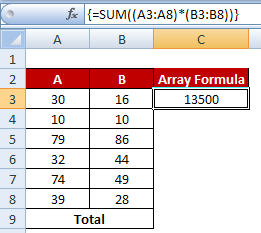Array Formula Examples
=SUM((A3:A8)*(B3:B8)) is equivalent to [(A3*B3)+ (A4*B4)+....+ (A8*B8)]
Hit CTRL SHIFT ENTER to confirm =SUM((A3:A8)*(B3:B8)). If you hit it correctly, you would see the formula wrapped in curley brackets {}.
Example II

Suppose you are asked to maximum value based on multiple criteria. The data are shown below -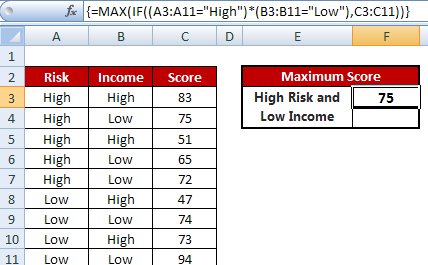Maximum Value based on Multiple Conditions
=MAX(IF((A3:A11="High")*(B3:B11="Low"),C3:C11))
Example III

Suppose you are asked to find out the top3 scorers in a class given the students can have same score.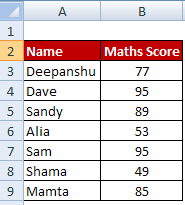Array Formulas Examples
=INDEX(\$A\$3:\$A\$9,MATCH(LARGE(\$B\$3:\$B\$9-ROW(\$B\$3:\$B\$9)/10^5,ROWS(A\$1:A1)),\$B\$3:\$B\$9-ROW(\$B\$3:\$B\$9)/10^5,0))

1. INDEX- MATCH looks for a value in the right column of a table and returns a value in the same row from a column you specify.

2. MATCH always return the first occurrence so we first need to create unique values in range.
\$B\$3:\$B\$9-ROW(\$B\$3:\$B\$9)/10^5
See how the above formula evaluates -
B3 - ROW(B3)/10^5 = 77-(3/100000)
B4 - ROW(B4)/10^5 = 95-(4/100000)
.
.
B9 - ROW(B9)/10^5 = 85-(9/100000)

It gives us unique values -
(76.99997, 94.99996,88.99995,52.99994,94.99993,48.99992,84.99991)

Example IV

We need to extract UNIQUE names from the list.Extract Unique List
Enter the following formula in cell C3
=INDEX(\$A\$3:\$A\$9,MATCH(0,COUNTIF(\$C\$2:\$C2,\$A\$3:\$A\$9),0))

Example V : Sum of Digits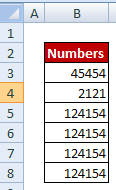Sum of Digits
=SUM(MID(B3,ROW(INDIRECT("1:" & LEN(B3))),1)*1)
The INDIRECT function returns a set of text values, in this case the values 1 through number of digits in a cell. The ROW function in turn generates a  multi-cell column array.
ROW(INDIRECT("1:" & LEN(B3)) evaluates to ROW(1:5) as the number of digits in cell B3 is 5.

Example VI : Count Uppercase and lowercase letters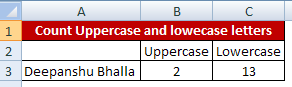Count Uppercase and Lowercase Letters
Count Uppercase letters
=SUMPRODUCT(LEN(A3)-LEN(SUBSTITUTE(A3,CHAR(ROW(INDIRECT("65:90"))),"")))
CHAR(ROW(INDIRECT("65:90"))) generates capital A through Z.

Count Lowercase letters
=SUMPRODUCT(LEN(A3)-LEN(SUBSTITUTE(A3,CHAR(ROW(INDIRECT("97:122"))),"")))
CHAR(ROW(INDIRECT("97:122"))) generates lowercase a through z.Related PostsDeepanshu founded ListenData with a simple objective - Make analytics easy to understand and follow. He has over 8 years of experience in data science. During his tenure, he has worked with global clients in various domains like Banking, Insurance, Telecom and Human Resource.

5 Responses to "Excel Array Formulas Examples"
1.Nice post. Never tried going into array formulas but this helped a lot to understand the basics of it.

1.Thank you for stopping by my blog. Cheers!

2.thanks my name amarnathverma@outlook.com

3.Thanks. Know I have got the better undersetting of Array.

4.in example 2, why are we dividing by 10 and raise it to 5?thanks

Next → ← Prev
Love this Post? Spread the Word!
Share